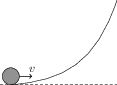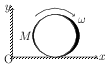# Rolling without Slipping of Rings, Cylinders and Spheres

## Problems from IIT JEE

Problem (IIT JEE 2007):A small object of uniform density rolls up a curved surface with an initial velocity $v$. It reaches up to a maximum height of $\frac{3v^2}{4g}$ with respect to the initial position. The object is,

1. ring
2. solid sphere
3. hollow sphere
4. disc

Solution: Let mass of the object be $m$, its radius be $r$, and moment of inertia about axis of rotation be $I$. We assume object to roll without slipping which gives, \begin{align} v=\omega r. \end{align} In rolling without slipping, work done by frictional force is zero and hence mechanical energy is conserved. Initial and final values of mechanical energy are, \begin{align} &K_i+U_i=K_i+0=\tfrac{1}{2}mv^2+\tfrac{1}{2}I\omega^2=\tfrac{1}{2}mv^2\left(1+\frac{I}{mr^2}\right),\\\ &K_f+U_f=0+U_f=mgh=mg\left(\frac{3v^2}{4g}\right)=\frac{3}{4}mv^2. \end{align} Equate initial and final mechanical energies to get $I=\frac{1}{2}mr^2$. This is moment of inertia of a disc about a normal axis passing through its centre.

Problem (IIT JEE 2002): A cylinder rolls up an inclined plane, reaches some height and then rolls down (without slipping throughout these motions). The directions of the frictional force acting on the cylinder are,

1. up the incline while ascending and down the incline while descending.
2. up the incline while ascending as well as descending.
3. down the incline while ascending and up the incline while descending.
4. down the incline while ascending as well as descending.

Solution:The force acting on the cylinder are weight $mg$ and normal reaction $N$. In the absence of frictional force, the component $mg\sin\theta$ will give an acceleration $g\sin\theta$ down the incline and hence contact point will have a tendency to move downward. To oppose this tendency, the frictional force will act upward. This is independent of whether cylinder is moving up or down. The readers are encouraged to show that friction reduces the acceleration to $g\sin\theta/\left(1+\frac{I}{mR^2}\right)$.

Problem (IIT JEE 1999):A disc of mass $M$ and radius $R$ is rolling with angular speed $\omega$ on a horizontal plane (see figure). The magnitude of angular momentum of the disc about the origin O is,

1. $\frac{1}{2}MR^2\omega$
2. $MR^2\omega$
3. $\frac{3}{2}MR^2\omega$
4. $2MR^2\omega$

Solution:The angular momentum of the disc about the origin O in combined rotation and translation motion is given by, \begin{align} \vec{L}_\text{O}=\vec{L}_\text{cm}+M\vec{r}_\text{C}\times\vec{v}_\text{C}.\nonumber \end{align} The first term $\vec{L}_\text{cm}$ represents the angular momentum of the disc as seen from the centre of mass frame. Thus, \begin{align} \vec{L}_\text{cm}=I\vec{\omega}=-\tfrac{1}{2}MR^2\omega\,\hat{k}, \end{align} where $I=\frac{1}{2}MR^2$ is moment of inertia of the disc about a perpendicular axis passing through C. The second term $M\vec{r}_\text{C}\times\vec{v}_\text{C}$ equals the angular momentum of the disc if it is assumed to be concentrated at the centre of mass translating with velocity $\vec{v}_\text{C}$. In pure rolling, velocity of the contact point P is zero. Thus, velocity of the centre of mass and the term $M\vec{r}_\text{C}\times\vec{v}_\text{C}$ are, \begin{align} &\vec{v}_\text{C} =\vec{v}_\text{P}+\vec{\omega} \times \vec{r}_\text{CP} = \omega R\,\hat\imath,\\ &M\vec{r}_\text{C}\times\vec{v}_\text{C}=M(x_0\,\hat\imath+R\,\hat\jmath)\times (\omega R\,\hat\imath)=-M\omega R^2\,\hat{k}. \end{align} Hence, angular momentum of the disc about the origin O is, \begin{align} \vec{L}_\text{O}=\vec{L}_\text{cm} + M\vec{r}_\text{C}\times\vec{v}_\text{C} = -\tfrac{3}{2}M\omega R^2\,\hat{k}.\nonumber \end{align}